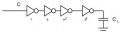# Inverter Buffers - Find CIn & CLoad, FanIn & FanOut

#### maximekahn

Joined Feb 15, 2020
1

#### Delta Prime

Joined Nov 15, 2019
1,311
Hello thereYou really have to know which logic family you're working with or combination of logic families for me it's easier for a chain of inverters to explain the calculations needed.
to drive load CL with optimum delay through the chain of inverters. Lets assume the input capacitance of first inverter is ‘C’ as shown in figure with unit width. Fanout being ‘a’ next inverter width would ‘a’ and so forth.
The number of inverters along the path can be represented as a function of CL and C like following.Total number of inverters along chain D = Loga(CL/C) = ln(CL/C)/ln(a)
Total delay along the chain D = Total inverters along the chain * Delay of each inverter. For a back to back inverters where driver inverter input gate capacitance is ‘C’ and the fanout ration of ‘a’, the delay through driver inverter is 3aRC
Total delay along the chain D = ln(CL/C)/ln(a) * 3aRC
The minimum value of total delay function for a specific value of fanout ‘a’, we need to take the derivative of ‘total delay’ with respect to ‘a’ and make it zero. That gives us the minima of the ‘total delay’ with respect to ‘a’.
D = 3*RC*ln(CL/C)*a/ln(a)
dD/da = 3*RC* ln(CL/C) [ (ln(a) -1)/ln2(a)] = 0
For this to be true
(ln(a) -1) = 0
Which means : ln(a) = 1, the root of which is a = e.